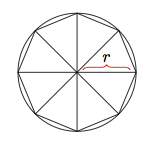# Area of a circle

Alignments to Content Standards: G-GMD.A.1

The goal of this task is to explain why the area enclosed by a circle $C$ of radius $r$ is $\pi r^2$. Recall that $\pi$ is the ratio of the circumference of a circle to its diameter and that this ratio is independent of the size of the circle.

1. Draw a picture of a regular octagon $O$ inscribed in $C$. Find a formula for the area of the octagon in terms of its perimeter.

2. Reasoning as in part (a), find a formula for the area of a regular $n$ sided polygon, for $n \geq 3$, inscribed in $C$: the formula should give the area of the polygon in terms of its perimeter.

3. Using your formula from part (b), explain why the area of $C$ is $\pi r^2$.

## IM Commentary

This purpose of this task is to develop an understanding of the formula for the area of the circle. The argument presented is essentially due to Archimedes. Strictly speaking, what is established in the argument is that the area of $C$ is at least $\pi r^2$. A similar argument can be applied to regular polygons circumscribing $C$ to show that the area of $C$ is at most $\pi r^2$. Alternatively, a more delicate argument can be used to show that the difference between the area of $C$ and the area of an inscribed regular $n$ sided polygon approaches zero as $n$ grows. The level of the solution is appropriate as the standard G-GMD.1 asks for an ''informal argument for the formulas for the circumference of a circle, area of a circle.'' Successful completion of this task may take substantial time and guidance and it is well suited for group work.

The teacher may wish to emphasize in parts (a) and (b) that students do not need to calculate the area of a regular octagon or a regular $n$-sided polygon: the formula can involve an unknown. This is important because the precise formula will involve trigonometric functions which are difficult to evaluate without a calculator and which do not necessarily shed any light on Archimedes' fundamental insight.

This standard of mathematical practice most closely aligned with this task is MP8, Look For and Express Regularity in Repeated Reasoning. In part (a) students must recognize that the sides of the regular octagon are all congruent in order to deduce a simple formula. This same reasoning then needs to be extended in part (b) providing a general formula for the area of a regular polygon with $n$ sides in terms of its perimeter. There is also an opportunity to support MP5, Use Appropriate Tools Strategically: if polar graph paper is available, students can readily inscribe a 12, 24, or even 36 sided polygon in the circle. This will help them to visualize why the altitudes of the triangles making up the regular polygon get closer and closer to the radius of the circle as the number of sides increases. They will also be able to visualize the area of these polygons getting closer and closer to the area of the circumscribed circle.

## Solution

1. Below is a picture showing a regular octagon whose vertices all lie on a given circle of radius of $r$:To find a formula for the area of this octagon, note first that the 8 triangles in the picture are all congruent: this follows, for example, from SAS since each triangle has two legs which are radii of the circle and all eight interior angles measure 45 degrees because the octagon is regular. Denoting one of these triangles as $T$, the area of the octagon is $8T$.

To find the area of one of the triangles, we draw a new picture (without the circle) showing an altitude of one of the triangles:The area of $T$ is $\frac{1}{2} \text{base} \times \text{height}$. In the picture, the base is $b$ and the height is $a$. So the area of the regular octagon is

\begin{align} 8 \times \text{Area}(T) &= 8 \times \left(\frac{1}{2} b \times a\right)\\ &= \left(8 \times b \right) \times \frac{a}{2} \\ &= \text{Perimeter(Octagon)} \times \frac{a}{2}. \end{align}

2. We can decompose our regular polygon $P_n$ with $n$ sides into $n$ congruent triangles as in part (a). We denote one of these triangles by $T_n$. We can then duplicate the reasoning at the end of part (a) to find the area of $P_n$. For this, we let $b_n$ denote the length of the base of $T_n$ and $a_n$ its height.

\begin{align} \text{Area}(P_n) &= n \times \text{Area}(T_n)\\ &= n \times \left(\frac{1}{2} b_n \times a_n\right)\\ &= \left(n \times b_n \right) \times \frac{a_n}{2} \\ &= \text{Perimeter($P_n$)} \times \frac{a_n}{2}. \end{align}
3. As the number of sides on our polygon $P_n$ increases, the perimeter of $P_n$ will approach the circumference of $C$ while the distance $a_n$ from the center of the circle to the sides of $P_n$ will approach the radius $r$ of $C$. Finally, the area of $P_n$ approaches the area of $C$. Putting all of this together we get $$\text{Area}(C) = \text{circumference}(C) \times \frac{r}{2}.$$ Since the circumference of $C$ is $2 \pi r$ this is the same as $$\text{Area}(C) = 2 \pi r \times \frac{r}{2} = \pi r^2.$$